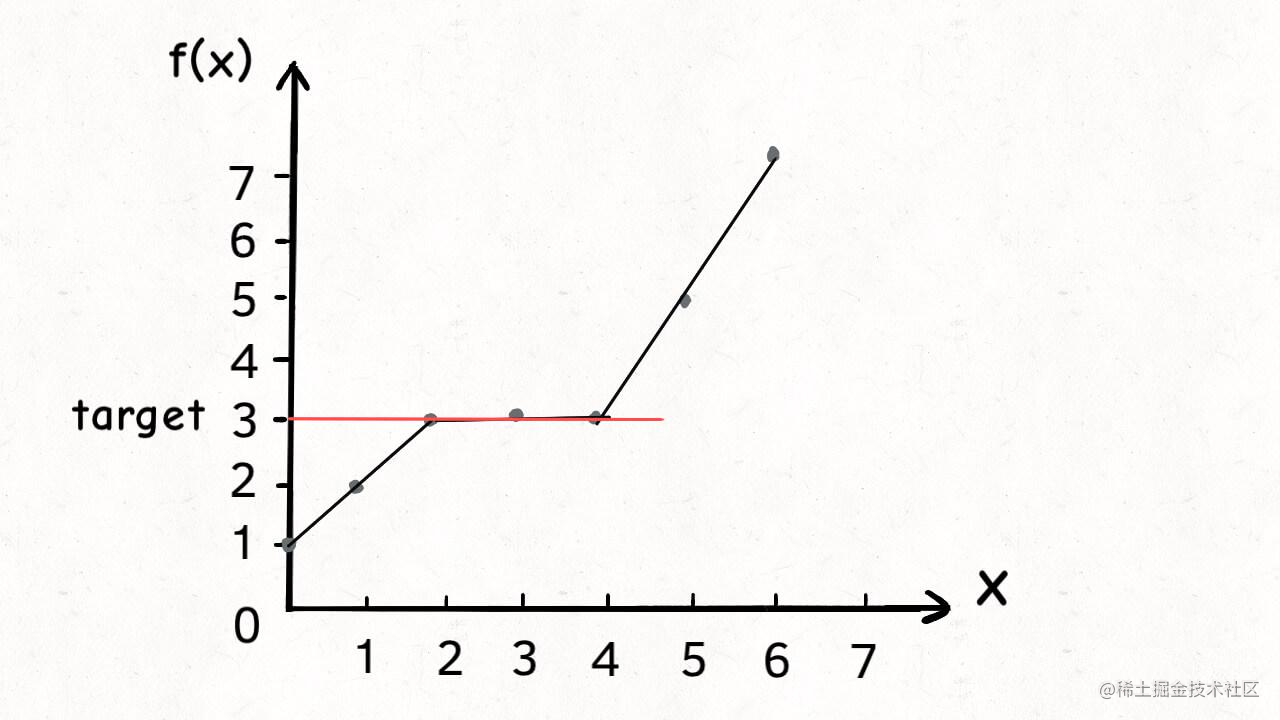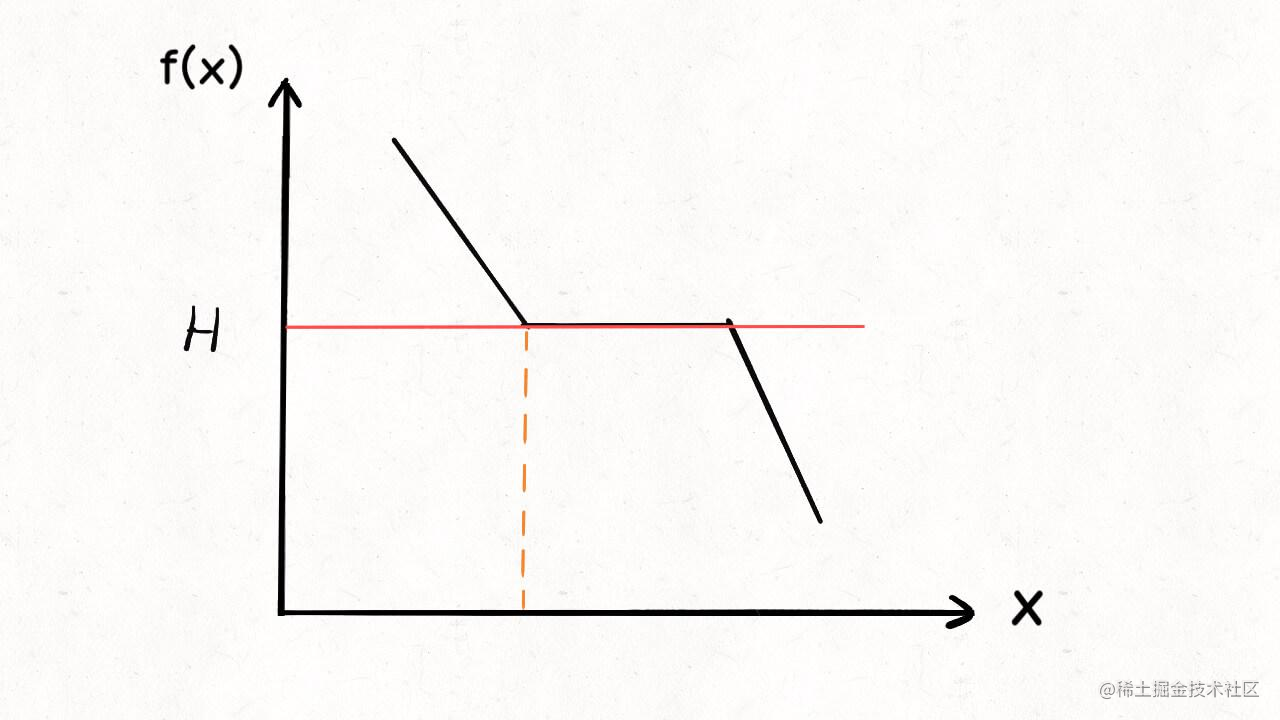# 一、題目描述：

1 <= piles.length <= 10^4 piles.length <= H <= 10^9 1 <= piles[i] <= 10^9

# 二、解題思路

## 2.1 遞增和遞減函式## 2.2 解題

### 2.2.1. 確定x

x就是 每小時吃香蕉的根數： x根/小時。

### 2.2.2 確定f(x)

``` int f(int[] piles,x){ int hours=0; for(int i=0;i0){ hours++; } } return hours; }

```

### 2.2.3.確定約束條件

target==H。相當於找到等於target的元素。

### 2.2.4 左右邊界確定

left左邊界明顯為1,有邊界，看題目描述： 1 <= piles[i] <= 10^9，因此right取1000000000。當然，因為x表示吃香蕉的根數，它不會超過N堆香蕉的最大根數，所以可以遍歷取得piles[i]中的最大值。

```public int minEatingSpeed(int[] piles, int H) {     int left = 1;     int right = 1000000000 + 1;          while (left ‹ right) {         int mid = left + (right - left) / 2;         if (f(piles, mid) == H) {             // 搜尋左側邊界，則需要收縮右側邊界             right = mid;         } else if (f(piles, mid) ‹ H) {             // 需要讓 f(x) 的返回值大一些             right = mid;         } else if (f(piles, mid) › H) {             // 需要讓 f(x) 的返回值小一些             left = mid + 1;         }     }     return left; }```

``` class Solution { public int minEatingSpeed(int[] piles, int h) {

``````    int left=1;
int right=1000000000;
while(left<=right){
int mid=left+(right-left)/2;
int cost= eatCostTime(mid,piles);
if(cost> h){
//因為是遞減函式，所以要讓eatCostTime返回更小的值。因此調整的是左邊界
left=mid+1;
}else if(cost< h){
//因為是遞減函式，所以要讓eatCostTime返回更大的值。因此調整的是左邊界
right=mid-1;
}else{
System.out.println("mid: "+mid);
if(0==mid||eatCostTime(mid-1,piles)!=h){
return mid;
}else{
right=mid-1;
}

}

}
// 沒有找到
return left;

}

public int eatCostTime(int x,int[] piles){
int hour=0;
for(int i=0;i<piles.length;i++){
hour+=piles[i]/x;
if(piles[i]%x>0){
hour++;
}
}
return hour;
}
``````

}

```